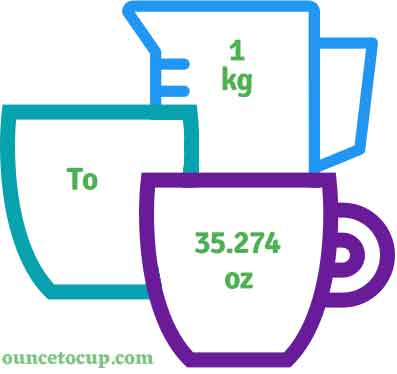# Kilogram to Ounce (kg to oz conversion)

Are you cooking your favorite dish? The detailed chart in the recipe includes the calculation of the 1 kilogram to ounce conversion.  Don't worry; use this calculator to determine how many 1 kilogram equals ounces in a minute.  This 1 kg to oz converter gives an exact measurement for any recipe you prepare.

Kilogram Value:

kg

Ounce Value:

oz

1 Kilogram = 35.274 Ounce
(1 kg = 35.274 oz)

Try our auto 1 Kilogram to Ounce Calculator (Without Convert Button), Just change the first field value and you got final value.## How many oz is a kg?

We know that the mass value of 1 kg is equal to 35.274 oz. If you want to convert 1 kg to an equal number of oz, just multiply the mass value by 35.274. Hence, 1 kilogram is equal to 35.274 oz.

The Answer is: 1 US Kilograms = 35.274 US Ounce

1 kg = 35.274 oz

Many of them try to search or find an answer for what is 1 kilograms in oz? So, we’ll start with 1 kg to oz conversion to know how big is 1 kg.

## How To Calculate 1 kg to oz?

To calculate 1 kilograms to an equal number of ounce, simply follow the steps below.

Kilograms to Fluid Ounces formula is:

Ounce = Kilogram * 35.274

Assume that we are finding out how many oz were found in 1 fl kg of water, multiply by 35.274 to get the result.

Applying to Formula: oz = 1 kg * 35.274 = 35.274 oz.

## How To Convert kg to oz?

• To convert kilograms to oz,
• Simply multiply the kilogram value by 35.274.
• Applying to the formula, oz = 1 kilograms * 35.274 [1x35.274].
• Hence, 1 kilograms is equal to 35.274 oz.

## Some quick table references for kilogram to ounce conversions:

Kilogram [kg]Ounce [oz]
1 kg35.274 oz
2 kg70.548 oz
3 kg105.822 oz
4 kg141.096 oz
5 kg176.37 oz
6 kg211.644 oz
7 kg246.918 oz
8 kg282.192 oz
9 kg317.466 oz
10 kg352.74 oz
11 kg388.014 oz
12 kg423.288 oz
13 kg458.562 oz
14 kg493.836 oz
15 kg529.11 oz

## Reverse Calculation: How many kilograms are in a oz?

• To convert 1 oz to kg,
• Simply divide the 1 oz by 35.274.
• Then, applying the formula, kg = 1 / 35.274 [1/35.274 = 0.0283495].
• Hence, 1 oz is equal to 0.0283495 kg.

### Related Converter:

Formula: kilogram to oz

oz = kilogram * 35.274

Applying to Formula,

oz = 1*35.274 = 35.274

1 kg = 35.274 oz